## Abstract

This paper presents unsteady numerical results of double diffusive mixed convection flow in a trapezoidal enclosure with the uniform magnetic field effect applied in negative horizontal direction. At the bottom wall, the uniform and non-uniform heat and mass are applied while the heat and mass absorbed uniformly at the top wall. Other side walls are impermeable and adiabatic. The top wall moves along x-axis direction with a constant velocity. The transport phenomenon of this problem can be expressed by the coupled governing equation derived from the conservation of mass and momentum along with the energy equation for temperature and concentration. The finite element method (FEM) based on Galerkin weighted residual technique is used to compute the numerical result from these governing equations. The numerical computation is carried out for Lewis number (Le = 0.1–50) and Richardson’s number (Ri = 0.1–100). Computed numerical results of mass, temperature and velocity distribution are expressed graphically as iso-concentration lines, isotherm lines and streamlines respectively. Average Sherwood and Nusselt number values are used to show the mass and heat transfer rate from the heated and concentrated surface of the enclosure. It is found from the analysis that mass transfer strongly depends on Lewis number. Heat and mass transfer for uniformly heated and concentrated bottom wall is larger than the non-uniformly heated and concentrated bottom wall. Finally, a correlation has been done for average Nusselt and Sherwood numbers for both of the cases.

## Keywords

Double diffusive; Trapezoidal; FEM; Magnetic field; Mixed convection

## Nomenclature

B0- strength of magnetic field (Tesla)

Br- buoyancy ratio

c- concentration of species (kg m−3)

ch- high species concentration (kg m−3)

cL- low species concentration (kg m−3)

C- dimensionless species concentration

D- species diffusivity (m2 s−1)

g- gravitational acceleration (m s−2)

Ha- Hartmann number

L- cavity height (m)

Le- Lewis number

Nu- Nusselt number

Pr- Prandtl number

Ri- Richardson number

Re- Reynolds number

Sh- Sherwood number

T- temperature (K)

Th- hot wall temperature (K)

Tl- cold wall temperature (K)

(uv)- velocity components (m s−1)

(UV)- dimensionless velocity components

U0- lid velocity (m s−1)

(xy)- dimensional coordinates (m)

(XY)- dimensionless coordinates

### Greek symbols

α- thermal diffusivity (m2 s−1)

βT- thermal expansion coefficient (K−1)

βc- compositional expansion coefficient (m3 kg−1)

μ- dynamic viscosity (kg m−1 s−1)

ν- kinematic viscosity (kg m−1 s−1)

θ- non-dimensional temperature

ρ- density

σ- electric conductivity (S m−1)

Ψ- dimensional stream function

ψ- dimensionless stream function

Ω- dimensional vorticity

ξ- dimensionless vorticity

${\textstyle {\nabla }^{2}}$- Laplacian operator

τ- dimensionless time

av- average

max- maximum

min- minimum

## 1. Introduction

The combined effect of temperature and concentration along with some external applied forces in which heat and mass transfer occur simultaneously is known as double-diffusive mixed convection. The considerable attention has been made mostly over the last decade to explore the double-diffusive mixed convection in lid driven enclosures. Several authors considered double-diffusive mixed convective heat and mass transfer inside the enclosure due to numerous applications in practical problem such as cooling of electrical equipment, nuclear reactor, transport of contaminant, geology and chemical process, and astrophysics and heat exchanger. Teamah et al.  considered lid driven rectangular enclosure to analyze the double-diffusive convection numerically. The working fluid is air. They showed the effect of Lewis number, Richardson number and buoyancy ratio on flow, temperature and concentration distribution inside the enclosure. With the increasing Richardson number, heat and mass transfer increase for both upper surface moves to left and right. On the other hand, mass transfer increase with the increase of Lewis number but heat transfer is not noticeable. However heat and mass transfer is more quickly for assisting flow than that of opposing flow. Laminar double diffusive mixed convection in a ventilated enclosure is studied by Deng et al.  for steady flow. The air indoor environment is investigated numerically with discrete heat and contaminant source. Numerical results depicted that the indoor air, contaminant and heat transport structures can be determined by the interaction between forced convection (mechanical ventilation) and natural convection (discrete heat/contaminant sources). The correlation between natural and forced convection is Re = 0.0125Gr + 272.41 at 103 ⩽ Gr ⩽ 3 × 104 and 300 < Re < 700. Besides two convection mechanisms play an important role on contaminant removal efficiencies. But Beya and Lili  considered unsteady double diffusive mixed convection in a ventilated enclosure as the continuation of the study of Deng et al. . The critical value of Grashof number is Gr = 2 × 105 relative to transition from steady to periodic for Re = 700. Flow remains periodic up to this value. The period of oscillations decreases with the increase of Gr whereas the main heat and mass transfer rate increases monotonously. The correlation between Reynolds number and Grashof number is Re = 11.285Gr0.338 for the onset of oscillations. Double diffusive convection plays an important role in crystal growth. It affects the fluid phase temperature and composition at phase interface since poor crystal quality is due to turbulence. Steady mixed convection in a lid driven square cavity due to the combined effect of thermal and mass diffusion was studied by Al-Amiri et al. . In their study, Galerkin weighted residual technique of finite element method is used to solve the transport equations. For a given range of Richardson number, their results depicted that higher heat and mass transfer rate can be attained. Mixed convective heat and mass transfer in various enclosures for different boundary condition has been found in the literature.

The flow of an electrically conducting fluid is influenced by magnetic force resulting from the interaction of induced electric currents with the applied magnetic field. An externally applied magnetic field is a widely used tool for the process of manufacturing metals. The convection inside the enclosure in the presence of magnetic field has been found in the literature for its wide range of application in industrial and engineering problem such as nuclear power plant, and crystal growth and chemical process. Chamkha and Al-Naser  investigated double-diffusive convective flow of a binary gas mixture in rectangular cavity in the presence of magnetic field. The numerical technique of finite difference method is used to solve the transport equation. No oscillatory behavior is viewed in the presence of magnetic field, but an oscillation is observed in the absence of magnetic field. However fluid circulation and heat transfer reduced in the enclosure with the increasing magnetic field. Oreper and Szekely  showed that the quality of crystal and the convective flow strongly depend on the presence of magnetic field. Melted silicon two dimensional natural convection is studied by Maruo  in the presence of magnetic field for the rate of heat transfer. The effect of transverse magnetic field on natural convection in a rectangular enclosure is investigated by Garandet et al.  and Alchaar et al. .

The plan of this study was to investigate the effects of Lewis number and Richardson number on double-diffusive mixed convection in a lid driven trapezoidal enclosure in the presence of magnetic field in terms of iso-concentration lines, isothermal lines, streamlines, both local and average Nusselt and Sherwood numbers when the bottom wall is uniformly and non-uniformly concentrated and heated.

## 2. Definition of considered model

Considered geometry of the physical model is depicted systematically in Fig. 1. The height of the enclosure is L and the corresponding length of the bottom and top wall is illustrated in the figure. Uniform and non-uniform heat and mass source is applied along the bottom wall while the top lid works as the sink of heat and mass. A magnetic field of strength B0 is applied along the negative x-axis direction and top lid moves along x-axis direction with the velocity U0. The inclined walls of the enclosure are adiabatic and impermeable. An electrically conduction fluid with Pr = 7.0 filled the enclosure to get the effect of magnetic field.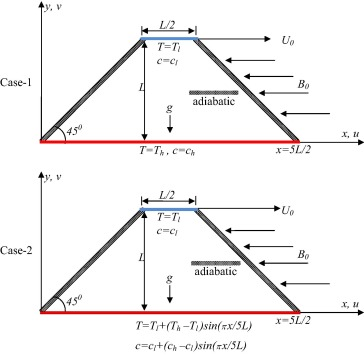Figure 1. Schematic diagram for the problem with boundary conditions.

## 3. Governing equations and numerical solution

It is considered that the enclosure is filled with Newtonian fluid. To valid the Boussinesq approximation, constant properties of fluid are assumed. Radiation mode of heat transfer, magnetic induction and viscous dissipation effect are neglected. The governing equations based on these assumptions are couple equations of momentum and energy. The energy equations are considered for temperature and concentration. The non-dimensional forms of the governing equations are given below:

 ${\frac {\partial \xi }{\partial \tau }}+U{\frac {\partial \xi }{\partial X}}+$$V{\frac {\partial \xi }{\partial Y}}={\frac {1}{Re}}{\nabla }^{2}\xi +$$Ri\left(-{\frac {\partial \theta }{\partial X}}+Br{\frac {\partial C}{\partial X}}\right)-$${\frac {{Ha}^{2}}{Re}}{\frac {\partial V}{\partial X}}$
(1)

 ${\frac {\partial \theta }{\partial \tau }}+U{\frac {\partial \theta }{\partial X}}+$$V{\frac {\partial \theta }{\partial Y}}={\frac {1}{RePr}}{\nabla }^{2}\theta$
(2)

 ${\frac {\partial C}{\partial \tau }}+{\frac {\partial C}{\partial X}}+$$V{\frac {\partial C}{\partial Y}}={\frac {1}{LeRePr}}{\nabla }^{2}C$
(3)
 $\xi ={\frac {\partial V}{\partial X}}-{\frac {\partial U}{\partial Y}}=$$-{\nabla }^{2}\psi$
(4)

where the dimensionless variables are introduced as follows:

 $X={\frac {\left(x{\mbox{,}}y\right)}{L}}{\mbox{,}}U={\frac {\left(u{\mbox{,}}v\right)}{U_{0}}}{\mbox{,}}\psi =$${\frac {\Psi }{U_{0}L}}{\mbox{,}}\theta ={\frac {T-T_{l}}{T_{h}-T_{l}}}{\mbox{,}}C=$${\frac {c-c_{l}}{c_{h}-c_{l}}}{\mbox{,}}\xi ={\frac {\Omega L}{U_{0}}}{\mbox{,}}\tau =$${\frac {U_{0}t}{L}}$
(5)

In fluid mechanics, the variables have their usual meaning as depicted in the nomenclature. It can be seen from the Eqs. (1), (2) and (3) that the dimensionless parameters are Reynolds’s number (Re), Richardson number (Ri), Prandtl number (Pr), buoyancy ratio (Br), Lewis number (Le), and Hartmann number (Ha), which are defined as follows:

 $Re={\frac {U_{0}L}{\nu }}{\mbox{,}}Ri={\frac {g{\beta }_{T}(T_{h}-T_{l})L}{U_{0}^{2}}}{\mbox{,}}Pr=$${\frac {\nu }{\alpha }}{\mbox{,}}Br={\frac {{\beta }_{c}(c_{h}-c_{l})}{{\beta }_{T}(T_{h}-T_{l})}}{\mbox{,}}Le=$${\frac {\alpha }{D}}{\mbox{,}}Ha={\sqrt {\frac {\sigma }{\mu }}}B_{0}L$
(6)

The non-dimensional initial conditions are as follows:

 $U=V=\theta =C=\psi =0\quad \quad {\mbox{at}}\quad \quad \tau =$$0$

For τ > 0, the dimensionless boundary conditions are as follows:

 ${\mbox{On the inclined walls}}:\quad U=V=\psi ={\frac {\partial \theta }{\partial n}}=$${\frac {\partial C}{\partial n}}=0{\mbox{,}}\xi =-\left({\frac {{\partial }^{2}\psi }{\partial X^{2}}}+\right.$$\left.{\frac {{\partial }^{2}\psi }{\partial Y^{2}}}\right)$
 ${\mbox{On the top wall}}:\quad U=1{\mbox{,}}V=\theta =$$C=\psi =0{\mbox{,}}\xi =-{\frac {{\partial }^{2}\psi }{\partial Y^{2}}}$
 ${\mbox{On the bottom wall}}:\quad U=V=\psi =0{\mbox{,}}\xi =$$-{\frac {{\partial }^{2}\psi }{\partial Y^{2}}}\quad ({\mbox{a}})\quad C=$$\theta =1{\mbox{,}}\quad ({\mbox{b}})\quad C=\theta =sin\left({\frac {\pi X}{5}}\right)$

The local mass and heat transfer rates from the surface of concentration and heat sources are defined respectively as

 ${Sh}_{L}=-{\left({\frac {\partial c}{\partial Y}}\right)}_{Y=0}\quad \quad {\mbox{and}}\quad \quad {Nu}_{L}=$$-{\left({\frac {\partial \theta }{\partial Y}}\right)}_{Y=0}$

The average mass and heat transfer rates on the surface of contaminant and heat sources can be evaluated by the average Sherwood and Nusselt numbers, which are defined respectively as

 ${Sh}_{\mbox{avg}}=-{\int }_{0}^{1}{\frac {\partial c}{\partial Y}}dX$
(7)

and

 ${Nu}_{\mbox{avg}}=-{\int }_{0}^{1}{\frac {\partial \theta }{\partial Y}}dX$
(8)

The stream function is calculated from

 $U={\frac {\partial \psi }{\partial Y}}{\mbox{,}}V=-{\frac {\partial \psi }{\partial X}}$

Governing Eqs. (1), (2) and (3)driven from the conservation of mass, momentum and energy have been solved numerically using Galerkin weighted residual technique of finite element method. Accuracy of the computation can be increased either using fine mesh or using higher order elements. But the combination of higher order elements and fine mesh is an alternative way to get the accuracy and efficiency of the computation. That is why, the quadratic triangular elements are used to interpolate velocity and temperature for energy equations. On the other hand linear triangular element is implemented to interpolate the velocity and pressure for continuity and momentum equations.

The considered trapezoidal geometry of the physical model is discretized using quadratic triangular element consisting of 3200 element which is given in Fig. 2. Average Sherwood and Nusselt number is calculated with the changing of number of elements and no significant change is found.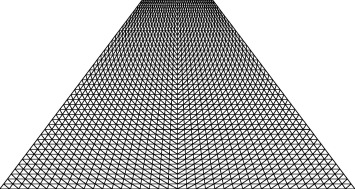Figure 2. Mesh of the trapezoidal enclosure considered in this work.

The test for validation of the current code was performed for the case of natural convection in a rectangular enclosure in the presence of magnetic field. The average Nusselt and Sherwood numbers using different Ha number have been compared with those obtained by Chamkha and Al-Naser  as shown in Table 1. A good agreement with the results was found.

Table 1. Comparison of Num and Shm with those of Chamkha and Al-Naser .
Ha Num Shm
Chamkha and Al-Naser  Present study Chamkha and Al-Naser  Present study
0 3.319 3.320 4.030 4.052
5 3.215 3.218 3.909 3.912
10 3.048 3.187 3.797 3.800
15 2.868 2.879 3.744 3.752
25 2.497 2.500 3.652 3.681

## 4. Result and discussion

A numerical study has been performed through Galerkin weighted residual finite element method to analyze the laminar mixed convection heat transfer and fluid flow in a lid driven cavity with uniformly and non-uniformly concentrated and heated bottom in the presence of magnetic field. In this section, average Nusselt and Sherwood number are calculated and represented in tabular form to demonstrate the heat and mass transfer rate for different time level. And then representative numerical results for the streamline, isotherm and iso-concentration contours of the cavity for various Lewis number Le (=0.1–50) and Richardson number Ri (=0.1–100) will be reported. The governing mixed convective parameter is Richardson number (Ri = Gr/Re2) which is used to analyze the relative importance of natural to forced convection. However the Lewis number measures the mass transfer exchange between the two different concentration zones. In addition, representative results for heat and mass transfer rate for the aforesaid parameters will be presented and discussed. In the present study the Prandtl number, Reynolds number, buoyancy ratio and Hartmann number are kept constant as Pr = 7.0, Re = 100, Br = 2 and Ha = 10 respectively.

Table 2, Table 3, Table 4 and Table 5 demonstrate the effect of time on heat and mass transfer for various values of Ri and Le in both cases. Average heat transfer rate is 13.8119 for τ = 0.1 and then sharply decreases and becomes 5.4848 for τ = 0.5 and then declines gradually and becomes 3.4939 for τ = 1.0 with increasing time in case-1 which are illustrated in Table 2. Similar observation is also seen in case-2 in which Nuav = 7.5471, 3.0049, 1.9380 for τ = 0.1, 0.5, 1.0 respectively. Average mass transfer rate quickly decreases initially and then decreases steadily with increasing time in both cases. For Ri = 0.1 and Le = 50, heat transfer rate almost remains same but exception is seen in case of mass transfer rate due to the higher value of Lewis number which are illustrated in Table 3. Shav = 109.7720, 32.5702 in case-1 whereas Shav = 60.0371, 17.9561 in case-2 for τ = 0.1, 1.0 respectively since mass transfer strongly depends on Lewis number. Almost similar observation is seen from Table 4 and Table 5 for Ri = 10. But heat and mass transfer rates are slightly higher than those for Ri = 0.1 due to the domination of buoyancy forces.

Table 2. Average Nusselt (Nuav) and Sherwood (Shav) numbers for different values of τ for Ri = 0.1 and Le = 0.1.
τ Nuav Shav
Case 1 Case 2 Case 1 Case 2
0.1 13.8119 7.7225 3.4899 1.9799
0.2 9.3905 5.1939 2.1074 1.1769
0.3 7.4349 4.1534 1.5496 0.8741
0.4 6.3088 3.5513 1.3004 0.7389
0.5 5.4848 3.0689 1.1643 0.6543
0.6 4.8661 2.7160 1.0927 0.6119
0.7 4.4001 2.4532 1.0598 0.5931
0.8 4.0369 2.2495 1.0468 0.5862
0.9 3.7431 2.0834 1.0427 0.5840
1.0 3.4939 1.9411 1.0406 0.5826

Table 3. Average Nusselt (Nuav) and Sherwood Shm numbers for different values of τ for Ri = 0.1 and Le = 50.
τ Nuav Shav
Case 1 Case 2 Case 1 Case 2
0.1 13.7119 7.5471 109.7720 60.0371
0.2 9.3116 5.2019 76.0034 41.9809
0.3 7.3785 4.1118 61.3360 33.7791
0.4 6.1940 3.4507 52.4685 28.8792
0.5 5.3909 3.0049 46.5149 25.6150
0.6 4.8153 2.6857 42.2777 23.3047
0.7 4.3748 2.4384 39.0465 21.5278
0.8 4.0278 2.2419 36.5040 20.1231
0.9 3.7394 2.0783 34.3880 18.9565
1.0 3.4925 1.9380 32.5702 17.9561

Table 4. Average Nusselt (Nuav) and Sherwood Shav numbers for different values of τ for Ri = 10 and Le = 0.1.
τ Nuav Shav
Case 1 Case 2 Case 1 Case 2
0.1 13.7030 7.6440 3.4568 1.9557
0.2 9.3082 5.3402 2.0832 1.2199
0.3 7.3365 4.2251 1.5170 0.8856
0.4 6.2269 3.6453 1.2655 0.7447
0.5 5.5042 3.2808 1.1553 0.6820
0.6 4.9770 3.0229 1.1013 0.6510
0.7 4.5760 2.8417 1.0681 0.6359
0.8 4.2933 2.7187 1.0505 0.6297
0.9 4.1151 2.6371 1.0468 0.6284
1.0 4.0107 2.5837 1.0303 0.6292

Table 5. Average Nusselt (Nuav) and Sherwood Shm numbers for different values of τ for Ri = 10 and Le = 50.
τ Nuav Shav
Case 1 Case 2 Case 1 Case 2
0.1 13.7094 7.6150 109.6608 60.4175
0.2 9.3121 5.2975 75.9718 42.5929
0.3 7.4239 4.1295 61.6608 33.8313
0.4 6.2654 3.5030 52.9985 29.1588
0.5 5.4870 3.0956 47.2196 26.1488
0.6 4.9039 2.7750 42.8929 23.7861
0.7 4.4482 2.5217 39.5060 21.9206
0.8 4.0848 2.3210 36.7864 20.4457
0.9 3.7916 2.1582 34.5639 19.2531
1.0 3.5520 2.0228 32.7091 18.2629

The effect of Lewis number on streamlines, isotherms and iso-concentrations for in case 1 at Ri = 0.1 is illustrated in Fig. 3. A large circular vortex is formed for all Le values with equal flow strength (Ψmin = −0.03) and occupying almost whole enclosure formed due to the domination of lid velocity. Forced convection domination mode takes place at Ri = 0.1 and the flow pattern is almost the same for all Le values at which the vortex rotates clockwise. A stagnant zone in the bottom corners of the enclosure is also observed. Overall, this means that the Le does not really have any effect on the streamlines for Ri = 0.1. The isothermal lines are almost similar for all Le values at which they are wavy near the top part of the cavity. Moreover, the isothermal lines are accumulated along the bottom wall of the cavity. Wavy shape isotherm lines formed below the right corner of the top lid due to the lid velocity and remain the same with the increase of Le since fluid flow almost remains the same for various values of Le. For (c), the cavity is fully occupied by the iso-concentrations contours for Le = 0.1. As Le increases to 1, the iso-concentrations at the top part of the cavity become wavy. The iso-concentrations start to gather along the bottom wall of the cavity for Le = 10 and eventually become thicker for Le = 50, which indicates higher mass transfer due to the depreciation of mass diffusivity.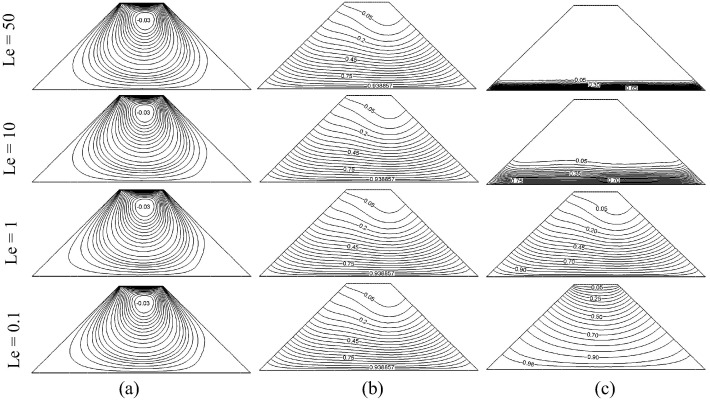Figure 3. Effect of Lewis number on (a) streamlines, (b) isotherms and (c) iso-concentrations for the case 1 and Ri = 0.1.

The effect of different values of Lewis number on streamlines, isotherms and iso-concentrations for case 2 for Ri = 0.1 is shown in Fig. 4. For (a), one large clockwise vortex at the center with two small counter clockwise vortices at the left and right side is formed for all Le values with equal flow strength. The two vortices at the sides become slightly smaller in size as Le increases. For (b), the isothermal lines are almost similar for all Le values at which they are wavy near the top and bottom left part of the cavity. Moreover, the isothermal lines are accumulated at the bottom right part of the cavity. For (c), the iso-concentrations contours are closely packed together at the top part of the cavity for Le = 0.1 but as Le increases to 1, the iso-concentrations at the top part start to become more wavy and sparse. The iso-concentrations contours start to accumulate at the bottom right corner of the cavity and eventually become thicker for higher Le values (Le = 10 and 50). Hence, there are no iso-concentrations contours appeared at the middle and top part of the cavity for higher Le values (Le = 10 and 50).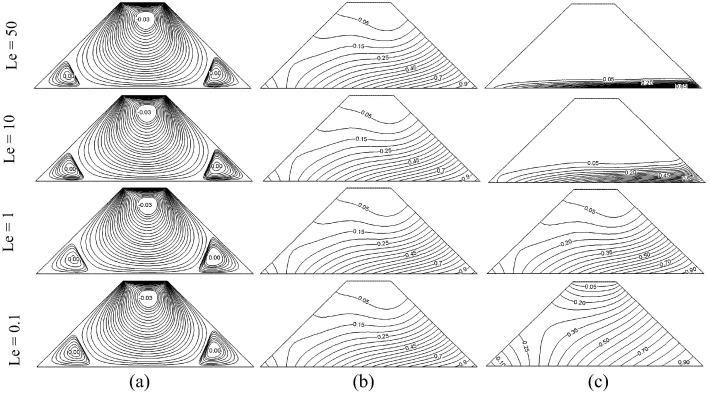Figure 4. Effect of Lewis number on (a) streamlines, (b) isotherms and (c) iso-concentrations for the case 2 and Ri = 0.1.

The effect of different values of Lewis number on streamlines, isotherms and iso-concentrations for case 1 for Ri = 1 is presented in Fig. 5. For (a), two vortices appeared for all Le values where one big clockwise vortex is at the center and the other smaller one is at the right at which it rotates in a counter clockwise direction. It can be seen that the smaller vortex reduces in size with the increase of Le. For (b) and (c), similar phenomena occurred as the isotherms and iso-concentrations observed in Fig. 3.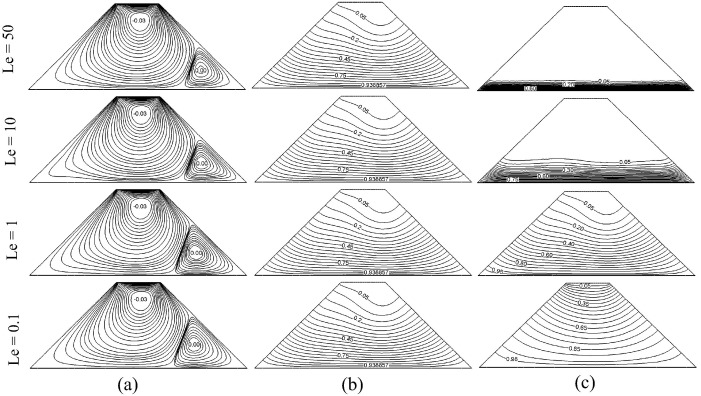Figure 5. Effect of Lewis number on (a) streamlines, (b) isotherms and (c) iso-concentrations for the case 1 and Ri = 1.

Fig. 6 presents the effect of different values of Lewis number on streamlines, isotherms and iso-concentrations for case 2 for Ri = 1. For (a), there are clockwise vortex located at the top and combined counter clockwise vortex located at the bottom for Le = 0.1. As Le increases to 1, the combined counter clockwise vortex at the bottom divides into two pieces and stayed at both left and right sides inside the cavity. The flow pattern almost remains the same as Le value increases to 10 except that the size of the vortices at the sides becomes slightly smaller and the vortex at the center grows slightly bigger. Drastic changes occurred for Le = 50; the vortices at the sides merge again into a two cellular single counter clockwise vortex and the size grows bigger than the clockwise vortex at the top. For (b) and (c), similar phenomena occurred as the isotherms and iso-concentrations observed in Fig. 4.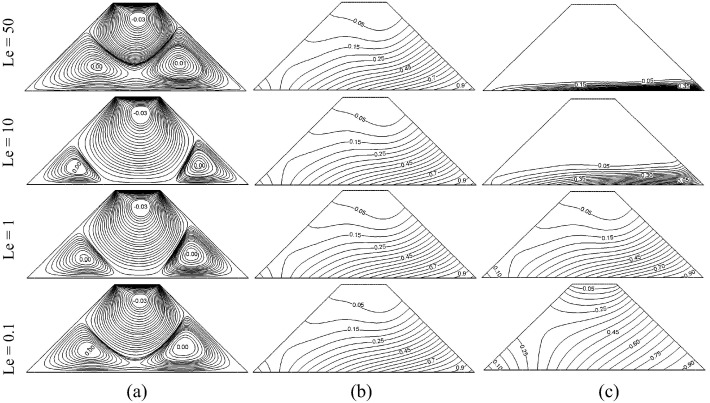Figure 6. Effect of Lewis number on (a) streamlines, (b) isotherms and (c) iso-concentrations for the case 2 and Ri = 1.

Fig. 7 shows the effect of different values of Lewis number on streamlines, isotherms and iso-concentrations for case 1 for Ri = 10. For (a), the flow pattern almost remains the same for all Le values except that the clockwise vortex becomes slightly bigger and the counter clockwise vortex becomes slightly smaller with the increase of Le. For (b), the accumulated isothermal lines along the bottom wall are thicker for lower Le values (Le = 0.1 and 1) and the thickness decreases for higher Le values (Le = 10 and 50). Moreover, the isothermal lines at the top part of the cavity are curvier for lower Le values (Le = 0.1 and 1) than the higher Le values (Le = 10 and 50). For (c), similar phenomena occurred as the iso-concentrations observed in Figure 3 and Figure 5 for Le = 0.1 and 50. As for Le = 1 and 10, it is observed that the contours are curvier than before.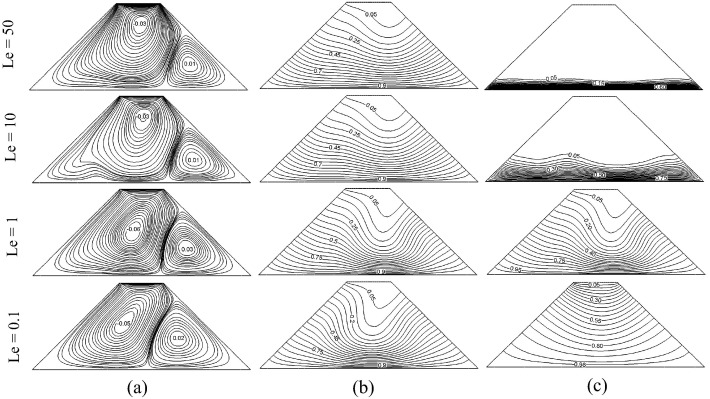Figure 7. Effect of Lewis number on (a) streamlines, (b) isotherms and (c) iso-concentrations for the case 1 and Ri = 10.

The effect of different values of Lewis number on streamlines, isotherms and iso-concentrations for case 2 for Ri = 10 is shown in Fig. 8. For (a), natural convection domination mode takes place for Le = 0.1 and 1. A big circular vortex is formed which rotates in a counter clockwise direction and a small clockwise vortex is formed at the top inside the enclosure for Le = 0.1. As Le increases to 1, the big counter clockwise vortex becomes slightly smaller in size and the clockwise vortex starts to grow bigger. On the other hand, the flow pattern and the flow strength are almost the same for Le = 10 and 50 where the counter clockwise vortex divides into two cellular single vortices and its size reduces whereas the clockwise vortex at the top becomes much bigger. For (b), the isothermal lines are the curviest for Le = 0.1 and it decreases with the increase of Le. For higher Le values (Le = 10 and 50), the isothermal lines are almost similar. For (c), similar phenomena occurred as the iso-concentrations observed in Figure 4 and Figure 6 for Le = 0.1 and 50. As for Le = 1 and 10, it is observed that the contours are slightly curvier than before.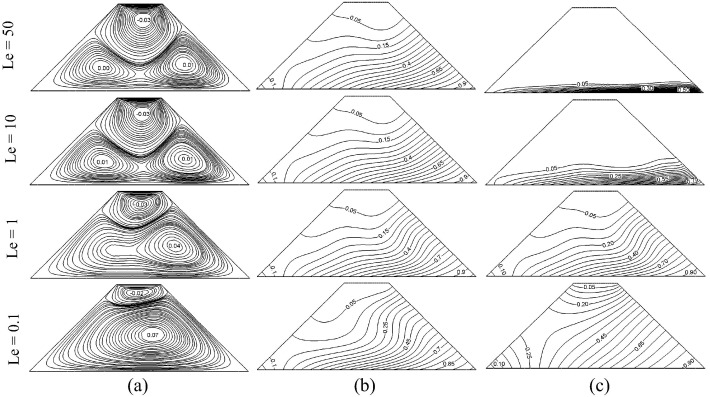Figure 8. Effect of Lewis number on (a) streamlines, (b) isotherms and (c) iso-concentrations for the case 2 and Ri = 10.

Fig. 9 presents the effect of different values of Lewis number on streamlines, isotherms and iso-concentrations for case 1 for Ri = 100. For (a), the flow pattern is almost the same for all Le values. As Le increases from 0.1 to 1, the stream function values for both clockwise and counter clockwise vortex decrease. As Le value increases to 50, the stream function value for the clockwise vortex increases again whereas the stream function value for the counter clockwise vortex remains the same. For (b), the accumulated isothermal lines along the bottom wall become thinner with the increase of Le. Overall, the isothermal lines are very wavy and the pattern is unstable for all Le values. For (c), the iso-concentrations contour becomes wavier and gradually spread with the increase of Le. In addition, the iso-concentrations contours that are accumulated along the bottom wall become extremely thicker as Le values increase.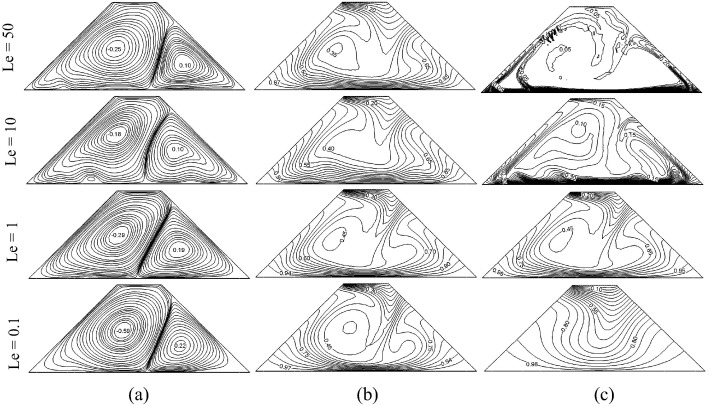Figure 9. Effect of Lewis number on (a) streamlines, (b) isotherms and (c) iso-concentrations for the case 1 and Ri = 100.

The effect of different values of Lewis number on streamlines, isotherms and iso-concentrations for case 2 for Ri = 100 is presented in Fig. 10. For (a), natural convection dominates for all Le values for Ri = 100 where a large circular counter clockwise vortex is formed. A very small vortex is formed at the top at which it rotates in a clockwise direction. The flow pattern is almost the same for all Le values. For (b), the isothermal lines have a circular pattern at the center of the cavity for Le = 0.1. As Le values increase, the isothermal lines become curvier with unstable pattern. For (c), the iso-concentrations contours become curvier with the increase of Le and it eventually settles along the bottom wall and along the right wall when Le = 50.Figure 10. Effect of Lewis number on (a) streamlines, (b) isotherms and (c) iso-concentrations for the case 2 and Ri = 100.

Fig. 11 shows the variation of local Nusselt number at different values of Lewis number for Ri = 100 for both cases. For (a), the graph of the local Nusselt number shows a stable parabolic shape for Le = 0.1, 1 and 50. Meanwhile for Le = 10, the graph shows a wavy pattern. For (b), the graph shown for the local Nusselt number is not as stable as (a). Maximum local Nusselt number is achieved for lowest Le value (Le = 0.1) for both cases.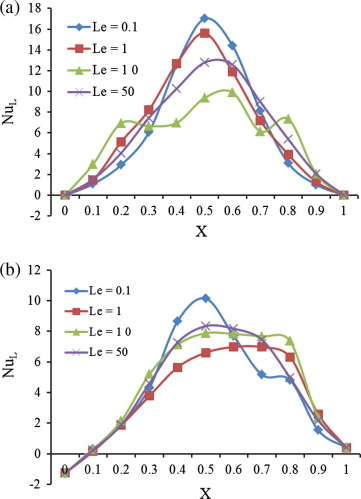Figure 11. Local Nusselt number for (a) case 1 and (b) case 2 at different values of Le for Ri = 100.

The effect of Lewis number on average Nusselt number with Richardson number for both cases is shown in Fig. 12. For (a), the average Nusselt number initially remains almost the same until it reaches Ri = 1 then it increases continuously for lower Le values (Le = 0.1 and 1); meanwhile, for higher Le values (Le = 10 and 50), it decreases slightly first then it starts to increase around Ri = 7. For (b), the average Nusselt number remains almost the same for Le = 0.1 until it reaches Ri = 1, then it starts to increase steadily. For Le = 1 and 10, the average Nusselt number initially remains almost the same until it reaches around Ri = 5, then it starts to continuously increase. On the other hand, for the highest Le value (Le = 50) the average Nusselt number increases slightly for Ri = 0.1 to 1 then it starts to decrease slightly until it reaches around Ri = 7, then finally it increases. Overall, higher heat transfer is observed in case 1 than in case 2.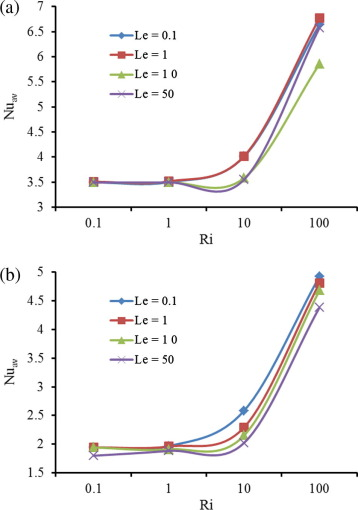Figure 12. Average Nusselt number versus Richardson number for (a) case 1 and (b) case 2 at different values of Le.

Fig. 13 presents the variation of local Sherwood number at different values of Lewis number for Ri = 100 for both cases. It can be seen that the maximum values of local Sherwood number decrease with decreasing of Le for both cases.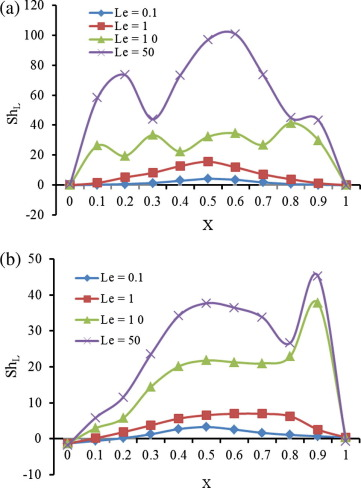Figure 13. Local Sherwood number for (a) case 1 and (b) case 2 at different values of Le for Ri = 100.

The effect of Lewis number on average Sherwood number with Richardson number for both cases is presented in Fig. 14. The average Sherwood number is stable and remains almost the same for Ri = 0.1 to 10, then for Ri = 10 to 100, it increases slightly for lower Le values (Le = 0.1 and 1) and rapidly for higher Le values (Le = 10 and 50) for both cases. Overall, higher mass transfer rate is achieved in case 1 compared to case 2.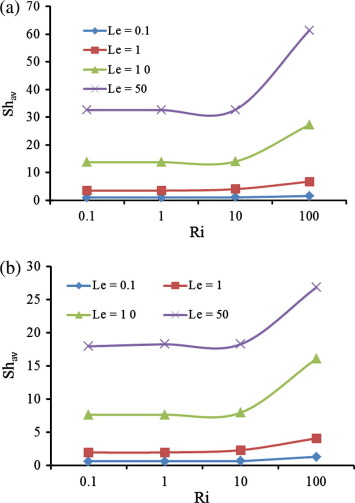Figure 14. Average Sherwood number versus Richardson number for (a) case 1 and (b) case 2 at different values of Le.

The relation between Nu and Sh is linearly correlated by the Nuav = 3.1857Shav + 1.9239 for case 1 and Nuav = 1.0967Shav + 5.414 for case 2.

## 5. Conclusions

The application of MHD on the characteristics of heat and mass transfer phenomenon is important because it has application in the metals and semiconductor industries to control the behavior of the melts during solidification processes including casting and crystal growth. The computational analysis has been carried out on unsteady double-diffusive mixed convection in a trapezoidal enclosure in the presence of magnetic field. The notable outcomes of this study are given below:

• Strength of circulation increases for higher values of Ri and decreases with the increase of Le in both cases but the number of cells diminishes for higher value of Ri in case-2.
• There has no significant effect of Le on temperature distribution up to Ri = 10. Closed isotherm line diminishes with the increase of Le for Ri = 100 which indicates the augmentation of heat transfer in both cases.
• Uniform iso-concentration lines have clustered along the bottom wall with the increase of Le up to the value of Ri = 10 and distorted with the increase of Le for Ri = 100 in both cases.
• Heat transfer rate is not significant for lower values of Ri but increases rapidly for higher value of Ri for all values of Le in both cases which shows the dependency of Ri on heat transfer.
• Mass transfer strongly depends on Lewis number.
• Heat and mass transfer is greater in case-1 than in case-2.
• Heat and mass transfer rate decreases monotonically with the increasing time in both cases.

### Document informationPublished on 12/04/17

Licence: Other

### Document Score0

Views 7
Recommendations 0